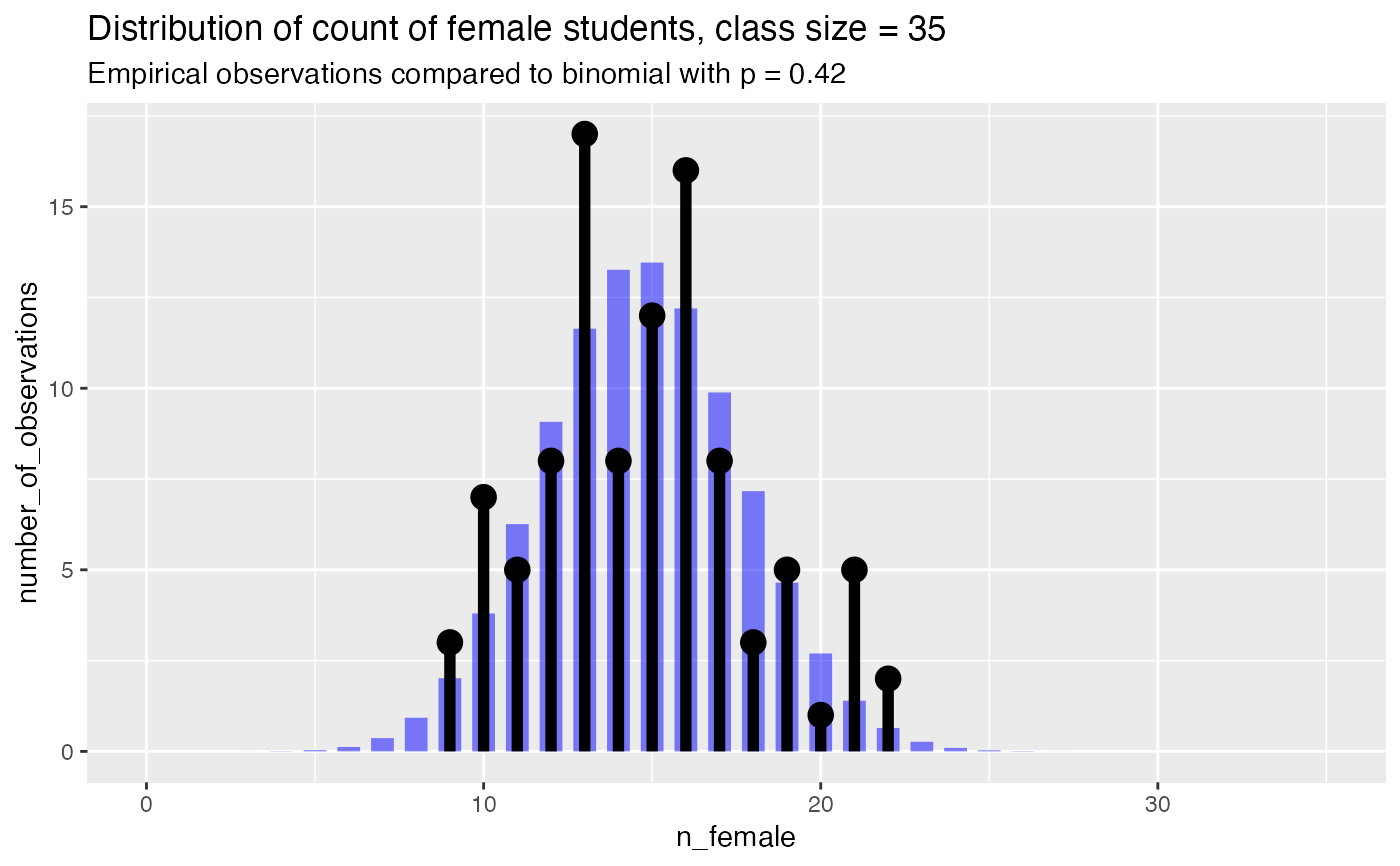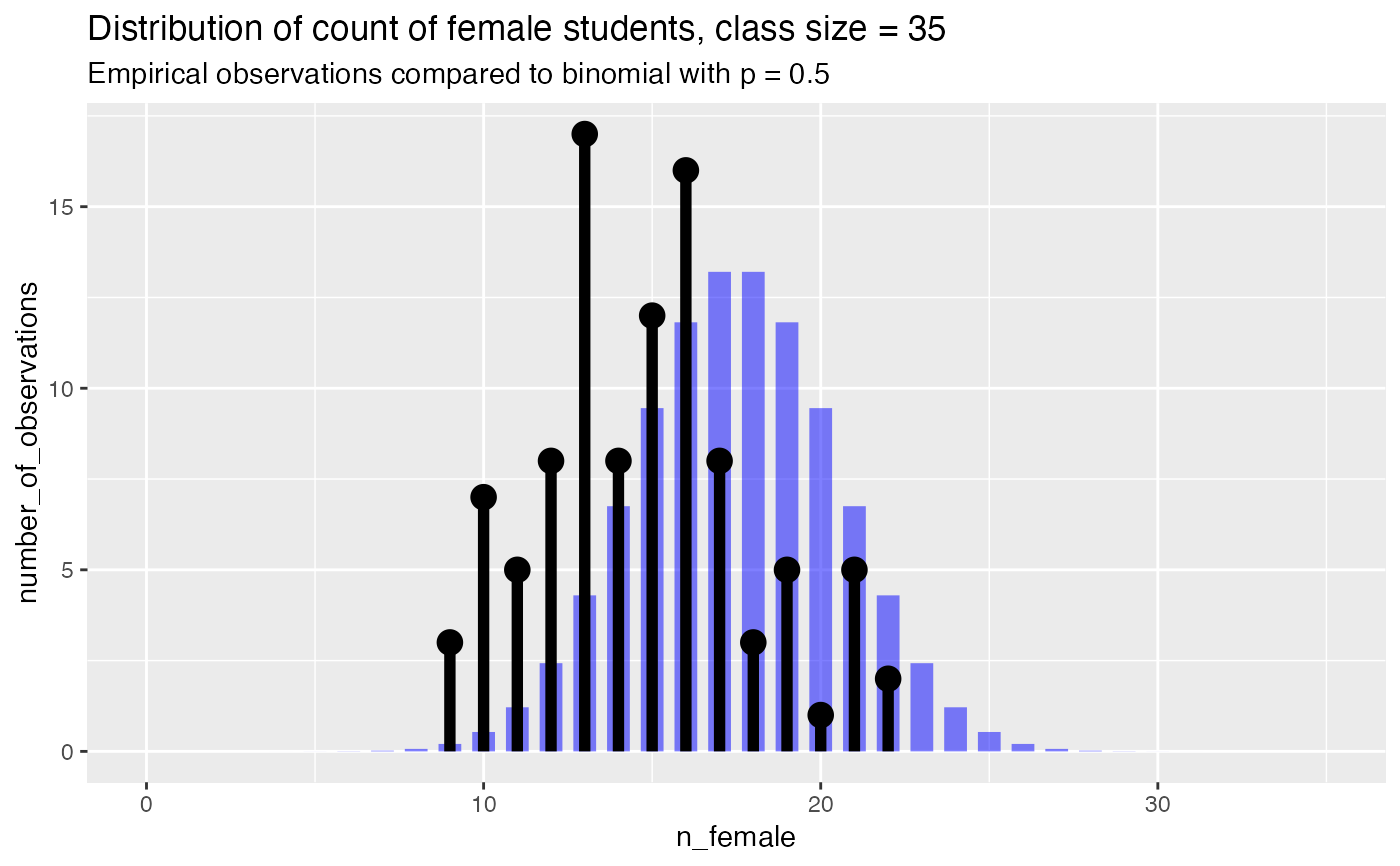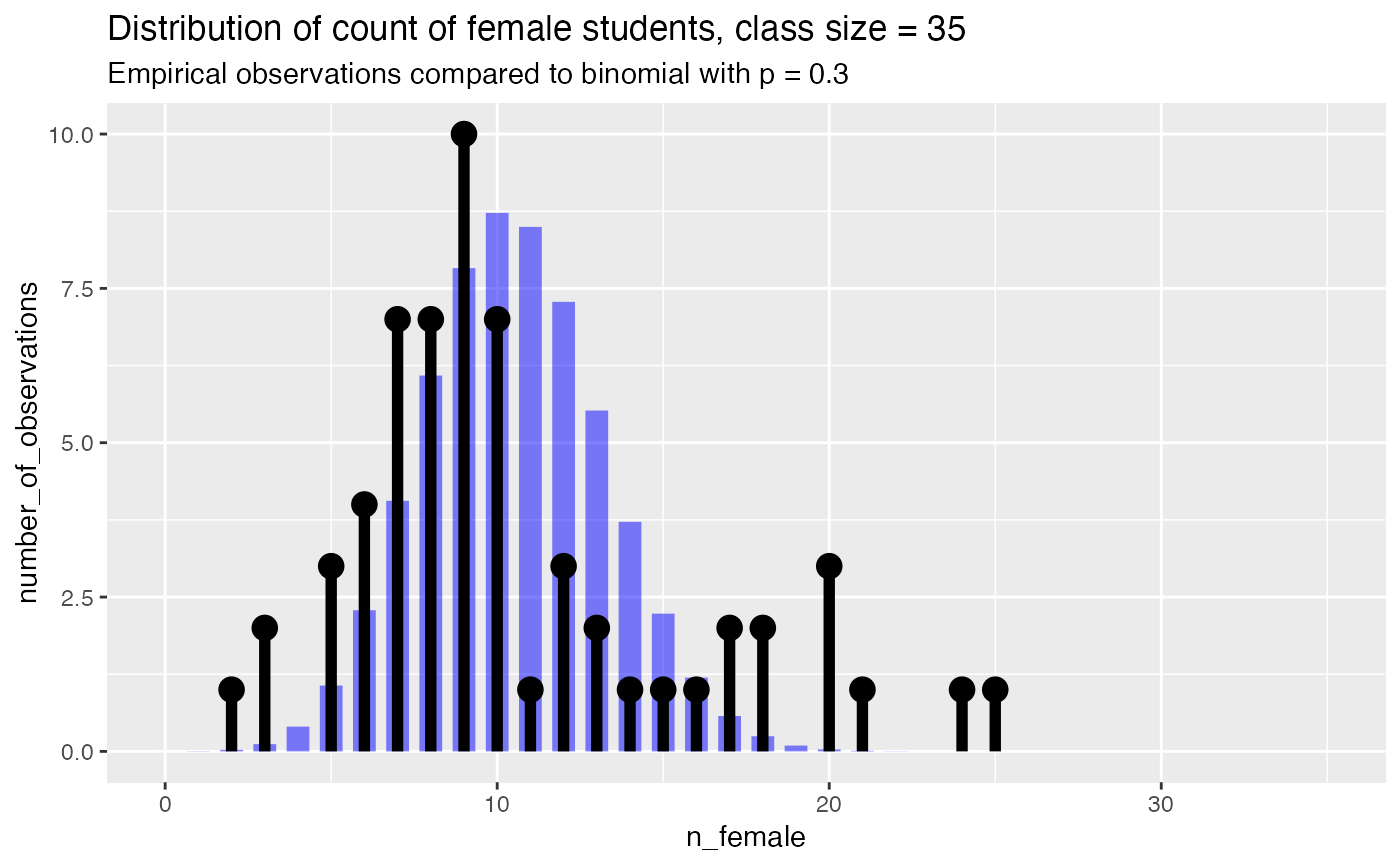Compares empirical count data to a binomial distribution

PlotDistCountBinomial(
frm,
xvar,
trial_size,
title,
...,
p = NULL,
limit_to_observed_range = FALSE,
count_color = "black",
binom_color = "blue"
)

## Arguments

frm data frame to get values from column of frm that counts the number of successes for each trial the number of "coin flips" in a trial title to place on plot no unnamed argument, added to force named binding of later arguments. mean of the binomial. If NULL, use empirical mean If TRUE, limit plot to observed counts color of empirical distribution color of theoretical binomial

## Details

This function is useful for comparing the number of successes that occur in a series of trials, all of the same size, to a binomial of a given success-probability.

Plots the empirical distribution of successes, and a theoretical matching binomial. If the mean of the binomial, p, is given, the binomial with success-probability p is plotted. Otherwise, p is taken to be the pooled success rate of the data: sum(frm[[xvar]]) / (trial_size*nrow(frm)). The mean of the binomial is reported in the subtitle of the plot (to three significant figures).

If limit_to_observed_range is TRUE, the range of the plot will only cover the range of the empirical data. Otherwise, the range of the plot will be 0:trial_size (the default).

PlotDistHistBeta, PlotDistDensityBeta,

## Examples

set.seed(23590)
class_size = 35
nclasses = 100
true_frate = 0.4
fdata = data.frame(n_female = rbinom(nclasses, class_size, true_frate), stringsAsFactors = FALSE)

title = paste("Distribution of count of female students, class size =", class_size)
# compare to empirical p
PlotDistCountBinomial(fdata, "n_female", class_size, title)# compare to theoretical p of 0.5
PlotDistCountBinomial(fdata, "n_female", class_size, title,
p = 0.5)# Example where the distribution is not of a true single binomial
fdata2 = rbind(data.frame(n_female = rbinom(50, class_size, 0.25)),
data.frame(n_female = rbinom(10, class_size, 0.60)),
stringsAsFactors = FALSE )
PlotDistCountBinomial(fdata2, "n_female", class_size, title)